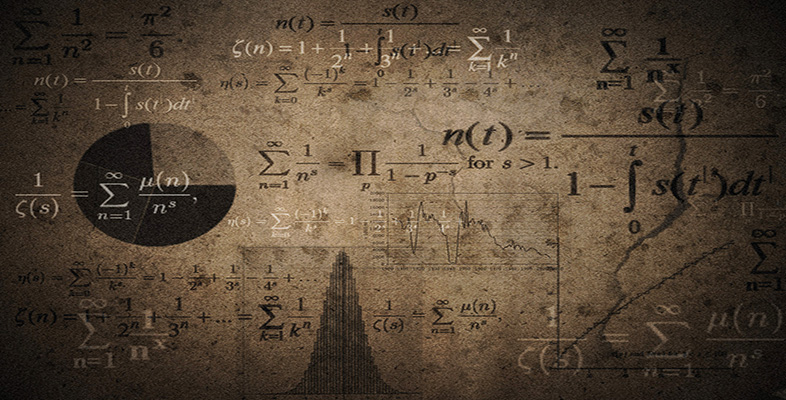Language, notation and formulas

Start this free course now. Just create an account and sign in. Enrol and complete the course for a free statement of participation or digital badge if available.

Free course

# 2.5 Vocabulary

In mathematics, some words are used in a more precise way than in English. It is important that a mathematical argument is unambiguous; therefore words that can be used in several contexts in English usually take only one meaning in mathematics. For instance, in English the word ‘sum’ might mean any calculation, but it has a precise mathematical meaning as exemplified by ‘The sum of 456 and 789 is 1245’. Similarly, in English the word ‘product’ can have a variety of meanings, but in mathematics the product of two numbers is the result obtained by multiplying those numbers together.

Below is a list of mathematical words and phrases, together with their mathematical meanings and examples of their use. You will have come across some of them before. Read them carefully and try to see the connections with the meanings that the words have in everyday English. You may well wish to copy the table into your notes and add to it in your future studies.

Word/phraseMathematical meaningExample of use
Even numberA whole number that is exactly divisible by two (that is, it results in a whole number when divided by two).432 is an even number.
Odd numberA whole number that is not exactly divisible by two.321 is an odd number.
SumThe addition of numbers.The sum of 123 and 456 is 579.
DifferenceThe numerical difference between two numbers, or the positive result of subtracting one number from the other.The difference between 24 and 42 is 18. The difference between 5 and 2 is 7.
ProductThe result of multiplying numbers together.The product of 3, 4 and 5 is 60.
QuotientOne number divided by another.The quotient 60 ÷ 12 is 5.
ExponentThe power to which a number is raised.In the expression (2.6)4, 4 is the exponent.
PerimeterThe outer boundary of a geometric figure (also the length of that boundary).The perimeter of the figure was drawn in red.
CircleA geometric figure in which every point on its perimeter is the same distance (the radius) from its centre.The circle has a radius of 4 cm.
EquationOne expression equaling another.The equation 9 − 6 = 3.
InequalityOne expression being less than or greater than another.3 < 6 is an inequality, which reads ‘3 is less than 6’.
MU120_4M6